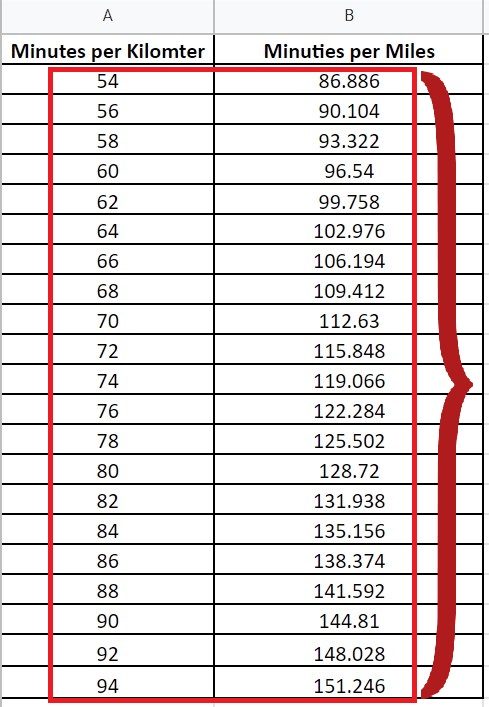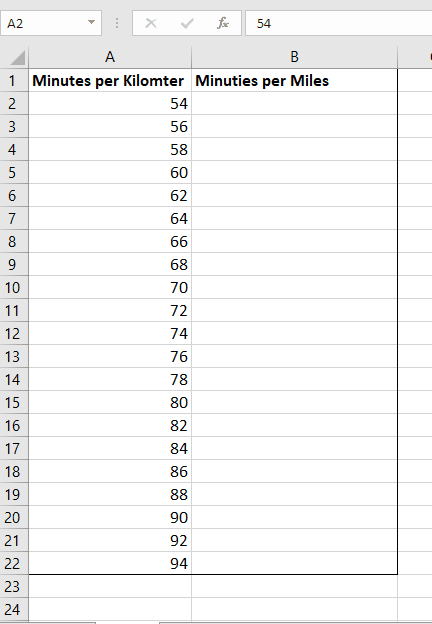# How to calculate a min per km to min per mile in Excel

One such requirement can be conversion of minutes per km to minutes per miles. Let’s start with an example. Suppose we have data in minutes per kilometers within a column and we have to convert all the values in that column to minutes per mile.

As we know that 1 Kilometer = 1.609 Miles so we’ll use this formula for conversion. However, the units of time on both sides are the same. So, we don’t need to convert it.

The following are the steps to follow.Excel is a data analysis and cleansing application. Excel enables users to organize and manage data in spreadsheets. In most cases, when we are working with data acquired from some scientific experiments, we face a situation where we have to do some scientific unit conversions.

### Step 1 – Select the cell and apply formula– Get any cell from the list of minutes per kilometer. In this case,  we selected the cell A2.

– Multiply the A2 value with 1.609. The formula will be;

=A2 * 1.609

– As long as you press the enter key, kilometers will be converted into miles.

– Drag it  down with the help of a handle, to convert the other values of  data range.